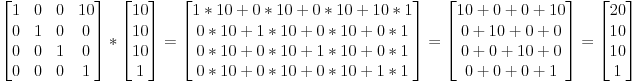Hello Guest

# What's the point of translating vertors is OpenGL wants matrices?

• 4 Replies
• 3392 Views####Mihai_Ionut_Floares

•• 43##### What's the point of translating vertors is OpenGL wants matrices?
« on: December 03, 2020, 17:36:39 »
I found this online and I don't understand why would we want to translate a vector:

"So if we want to translate the vector (10,10,10,1) of 10 units in the X direction, we get :"####KaiHH

•• 334##### Re: What's the point of translating vertors is OpenGL wants matrices?
« Reply #1 on: December 03, 2020, 17:53:01 »
The point of this tutorial http://www.opengl-tutorial.org/beginners-tutorials/tutorial-3-matrices/#translation-matrices is to illustrate how matrices work.
When using matrices practically in OpenGL, you are basically translating and scaling vectors _all the time_. It's just that it's not _you_ who does this, but the graphics card. You provide the GPU with matrices that translate, scale and rotate vertices. And the "position" component of a vertex is simply a vector, which is multiplied by the matrix. And if the matrix had a translational component to it, then that vector would get translated.####Mihai_Ionut_Floares

•• 43##### Re: What's the point of translating vertors is OpenGL wants matrices?
« Reply #2 on: December 03, 2020, 18:34:45 »
If GPU works with vectors why we(humans) need to work with matrices. It is a lot easier to work with vectors than matrices...####KaiHH

•• 334##### Re: What's the point of translating vertors is OpenGL wants matrices?
« Reply #3 on: December 03, 2020, 18:36:06 »
If GPU works with vectors why we(humans) need to work with matrices. It is a lot easier to work with vectors than matrices...
What?!
Matrices are a way to _encode_ transformations (translation, scaling, rotation) performed on vectors. Nothing more, nothing less.
If you want your models in the scene to show up somewhere else, be rotated a bit and scaled down, you _encode_ this information into a matrix, as simple as that.####Mihai_Ionut_Floares

•• 43##### Re: What's the point of translating vertors is OpenGL wants matrices?
« Reply #4 on: December 03, 2020, 22:27:33 »
Thank you